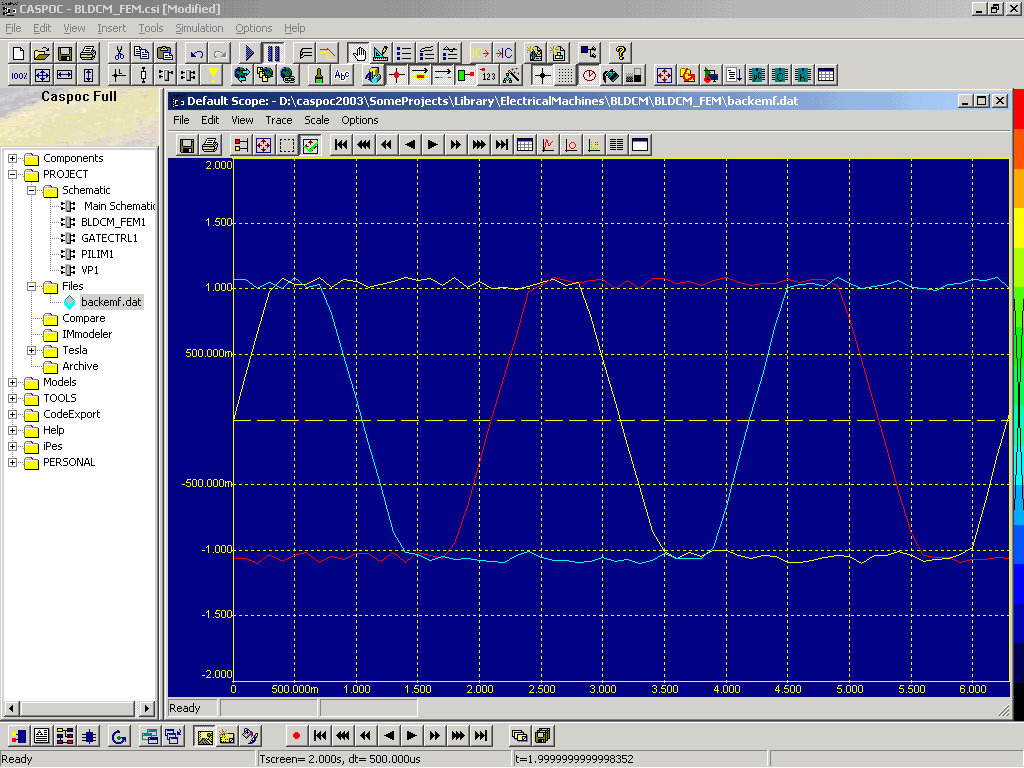• Introduction
• What is in this manual
• What is Caspoc
• User interface
• Introduction
• Starting
• Simulation
• Editing
• Viewing and printing
• Getting Started
• Basic editing
• Simulation in the time domain
• Basic User Interface Topics
• Editing
• Simulation
• Viewing
• Library
• Reports
• Project management
• Circuit and Block Diagram Components
• Introduction
• Cscript and user defined functions
• Component parameters
• Modeling Topics
• Introduction
• Power Electronics
• Semiconductors
• Electrical Machines
• Electrical drives
• Power Systems
• Mechanical Systems
• Thermal Systems
• Magnetic Circuits
• Green Energy
• Coupling to FEM
• Experimenter
• Analog hardware description language
• Embedded C code Export
• Coupling to Spice
• Small Signal Analysis
• Matlab coupling
• Tips and tricks
• Appendices

In the previous tutorials we looked at a DC Permanent Magnet motor. This tutorial will discuss a Permanent Magnet Synchronous Machine PMSM. We choose this example, because the equations are easy to understand and the structure does not get to complicate. First we will examine how to create a model in the two-phase ab frame, instead of using a three-phase system. We will use this principle for modeling our three-phase electrical machines, because most models are given either in the ab frame or in the dq frame.

Once the process of coupling the two-phase model with the three-phase electric circuit is understood, we will use this technique to create a model of a three-phase inductive load by using two-phase model in the ab frame. The model will be compared with a three-phase inductive load model. This model can then be used for creating other inductive loads, or electrical machine models.

For the second part of this tutorial we will look at the model for the PMSM that is build into the library of Caspoc. Here all equations are build inside the model, but we can change the parameters for this model. The reason that all equations are modeled into one block EM is that by combining as much as possible blocks into one block speeds up the total simulation time. We will extend the existing model of the PMSM with a look-up function to make the stator inductance current dependent.

The three-phase voltage is first transformed into the ab frame using a 3/2 transformation. The voltages Ua and Ub can be displayed in a scope and will rotate with the periodic frequency of the three-phase system. If a stationary frame is required, the ab-dq transformations can be used, where the angle of rotation has to be known. (This angle is mostly derived by integrating the angular speed with integrator block INTMOD with a build in modulus of 2 pi) Use the VOLTAGE blocks for reading the voltage from the electrical nodes. The nodes are defined at the controlled current sources that we use to feed back the resulting calculated current into the circuit.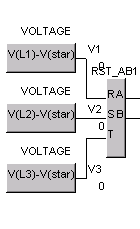If the results from our model are currents in the ab frame, we can transform them back into three-phase currents. If you do not want to export and connect the star node from your model, omit the third current source and export the third phase as the sum of the other two currents. Note that the labels of the nodes are the same as specified in he VOLTAGE blocks. Take care about the direction of the injected currents, the model should be passive, not active!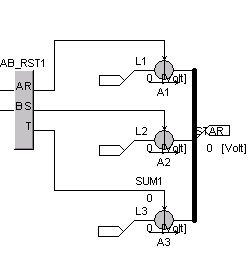The interface is ready. We can measure the voltage from the circuit, calculate the current and feed back the current into the circuit. The calculation part can be any model. Here we will discuss an inductive load. From the input voltage the product of current by resistance R is subtracted. The remaining voltage is then divided by the value of the inductance and finally integrated. The resulting current is the output from the equations. The model is displayed below where phase a is colored red and phase b is colored green. The resistor value is entered at the blue label while the inductor value is entered at the yellow label.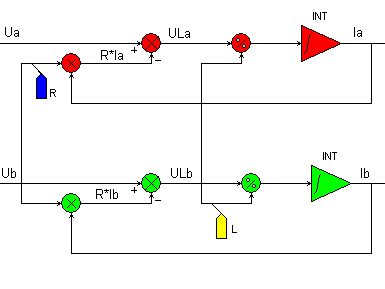If we combine all three models we get a three-phase inductive load based on a two-phase model.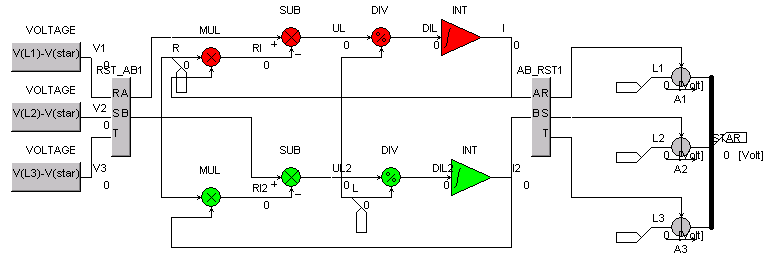The model is exported into one library model where the three-phase terminals are exported on he let side and the connections for defining R and L are exported on the bottom. The start terminal is exported on the right side. The model is connected to an inverter with 6-pulse block modulation and an output frequency of 50Hz. The voltage between node L1 and L3 and the current flowing into terminal L3 are measured and displayed in the scope. The star terminal is connected to ground via a dummy resistor of 1K. This keeps the star point from floating and ensures numerical stability during simulation.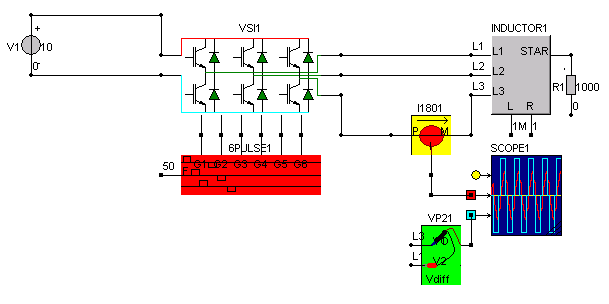The results from the simulation show clearly the resistive-inductive load.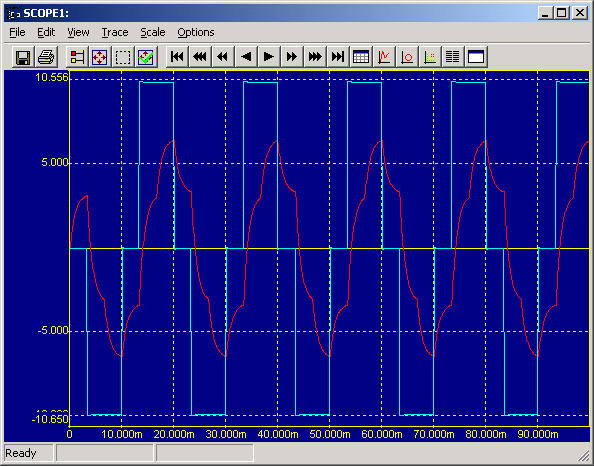Well that all looks nice, but is it correct? For that we compare the two-phase inductor model with a circuit model, where all three resistors and all three inductors are modelled. The resulting currents through the load for both models are compared and the error between them is also shown in a scope. The values for the load are R=1ohm and L=1mH.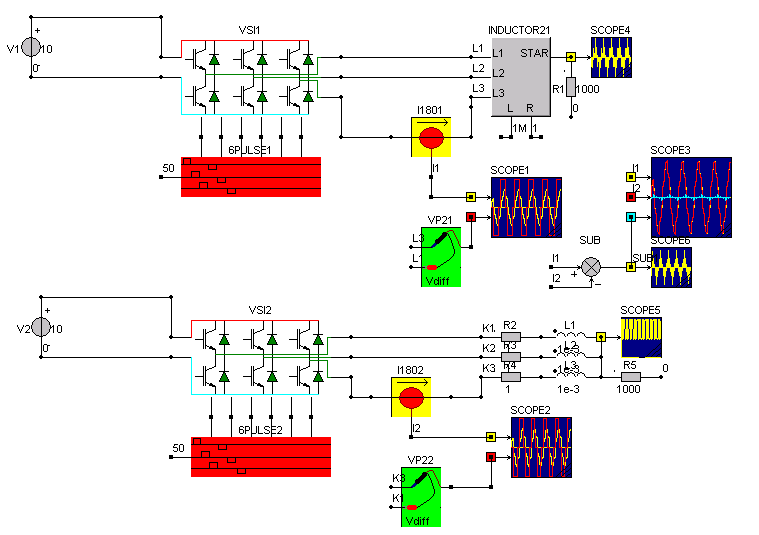In scope3 the currents flowing into terminal 3 are compared and the error between them is displayed as the blue trace. The two currents are displayed on top of each other in yellow and red.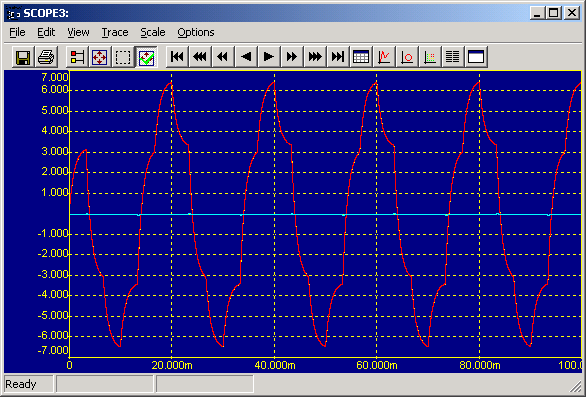To create faster models, use the modeling language instead of the block diagram. The modeling language could be compiled C code where you enter your equations. Also differential equations can be entered there.

For our resistive-inductive load example, the code could like like

static double R=1;

static double L=1e-3;

ua=(2*input-input-input)/3;

ub=(sqrt(3)*input-sqrt(3)*input)/3;

ia=x;

ib=x;

dxdt=(ua-ia*R)/L;

dxdt=(ub-ib*R)/L;

ir=ia;

is=(-ia+sqrt(3)*ib)/2;

it=(-ia-sqrt(3)*ib)/2;

bus=ir;

bus=is;

bus=it;

The first three inputs of the LibML are connected to the three voltage sources, while the three controlled sources are connected to three BUS blocks, that can read the variables from the bus[.] in the C code.

The array x[] and dxdt[] are used for creating differential equations.

The main advantage of the compiled C code is that the models are very compact and run very fast compared to a block diagram model. The drawback is that there is no animation, so you have to add watches if you want to debug the code. A watch is a variable that is copied into the bus[] array. Using the BUS block, you can see the value during the simulation in your block diagram.

Some of the electrical machine models that you will find in the library of Caspoc are based on the build in equations for the electrical machines. You will find the equations in the user guide. They are build into one block diagram model. In this section we will customize the model for the PMSM by making the inductance dependent on the inductor current.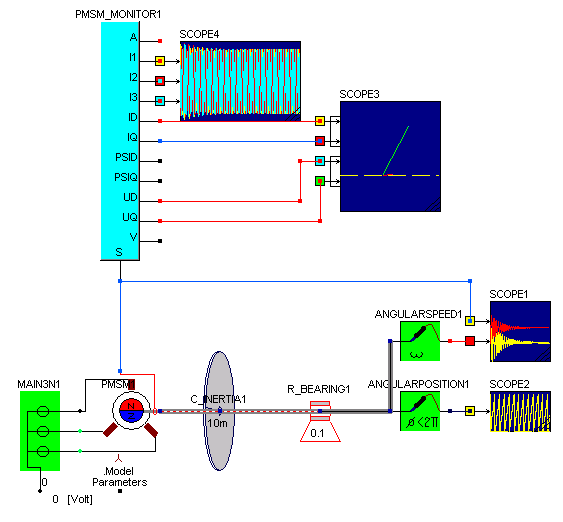The PMSM is directly coupled to the mains and will only synchronize for light loads. If we look at the model for the PMSM, we see that the model is build around the EM block.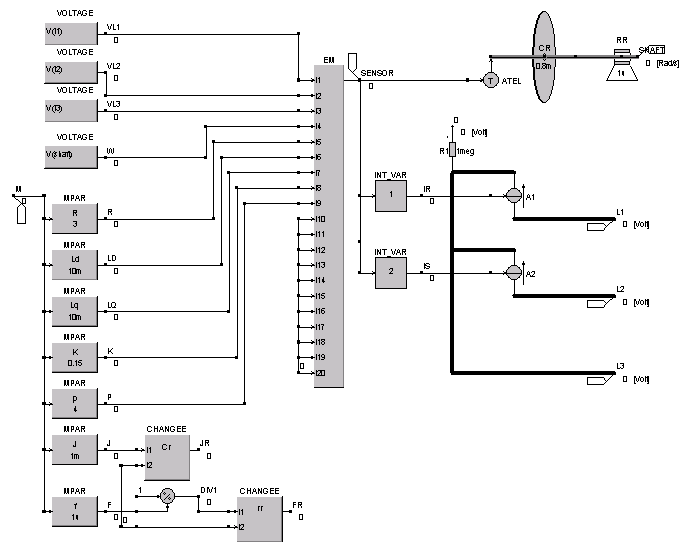The equations for this block are given below and can be found in the userguide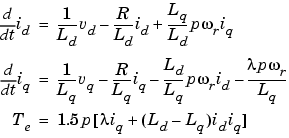If we would like to change the equations, we would have to build them again in either the blovk diagram or the modeling language as is explained in the first part ofthis tutorial. For now we are only interested in making the value of Ld and Lq dependent on the stator current. First we have to know the amplitude of the current. Since we are working with a threeepahse currents, a simple ABS block is not enough to get the magnitude of the current. Therefore we first transform the the three-phase currents into a two-phase system, from which we calculate the magnitude.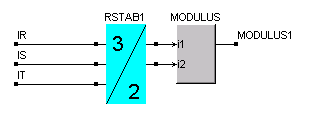The currents we get from the EM block and are exported from the EM block using the GetIntVar blocks.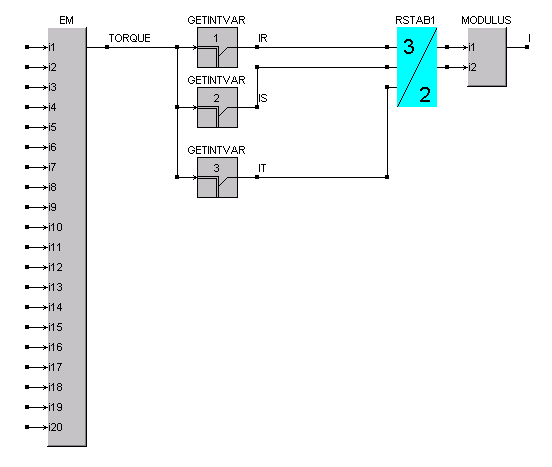The output from the modulus block is the magnitude of the three-phase current that is the index for our lookup table. The EM block is he EM block that is used in the machine model that we want to customize. The current dependent stator inductance is displayed below. Both Ld and Lq are calculated by using FEM and tabulated as function of the stator current.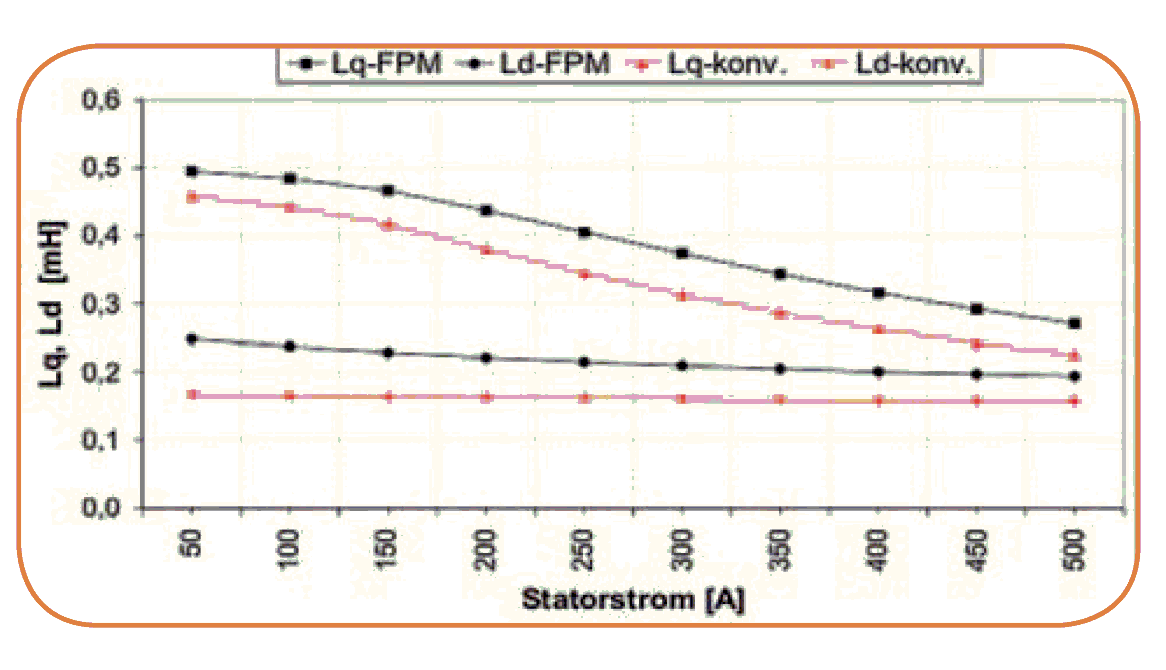The black traces are used in our example. We use a LookUp1D block to read the data and interpolate the values for Ld and Lq. From the PMSM model we can see that Ld is input number 6 and Lq is input number 7. Therefore we connect the output from the LookUp1D blocks to i6 and i7 of the EM block. The data for Ld and Lq can be stored in one ASCII data file. The first column is the current, the second column is Ld and the third column is Lq. In the LookUp1D block Lq we have to specify the we want o read the third column. (Default the second column is read.)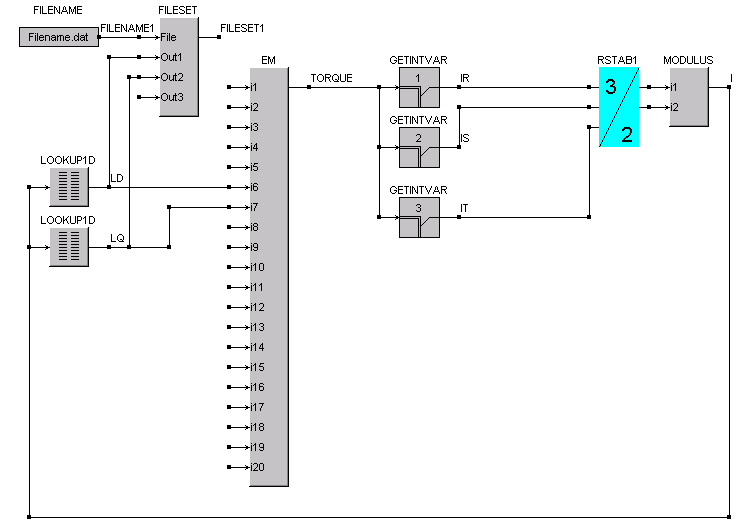The input from the FileSet block can be exported from the new PMSM machine library block. The user can connect the FILENAME block to specify the filename for the data file.

The same principle can be applied to the synchronous machine with external excitation.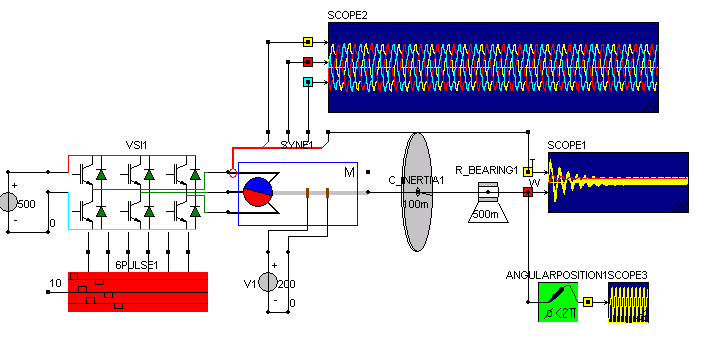If we open the block for the SYNE block, we see the same structure with MPAR blocks for specifying parameters and the EM block holding the equations.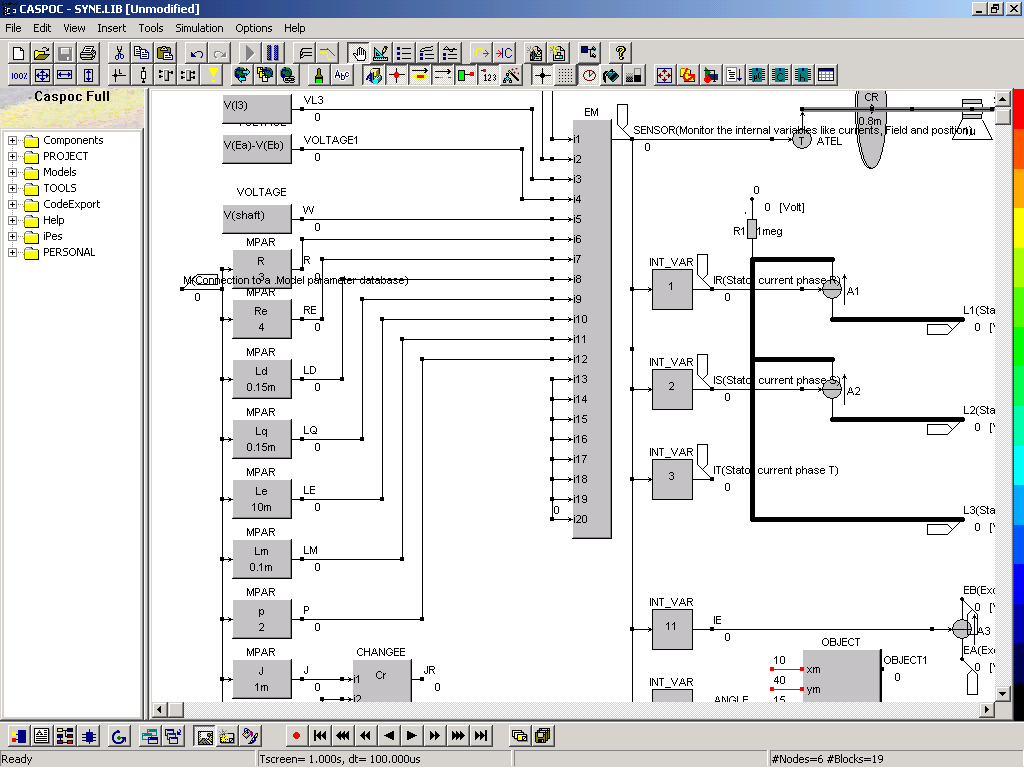For the SYNE block the inputs for Ld and Lq for the EM block are i8 and i9.

For modeling BLDCM machines, the back-emf can be calculated in FEM and used in the simulation. The back-emf of the BLDCM is calculated in FEM and for the three phases, the back-emf is stored in a data file. The BLDCM machine model is used in the following sample.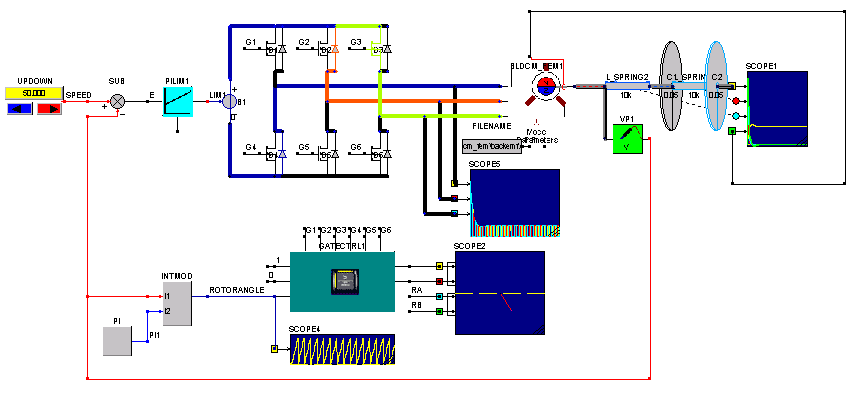If we open he BLDCM block we see that a circuit model is used for modeling the stator of the machine.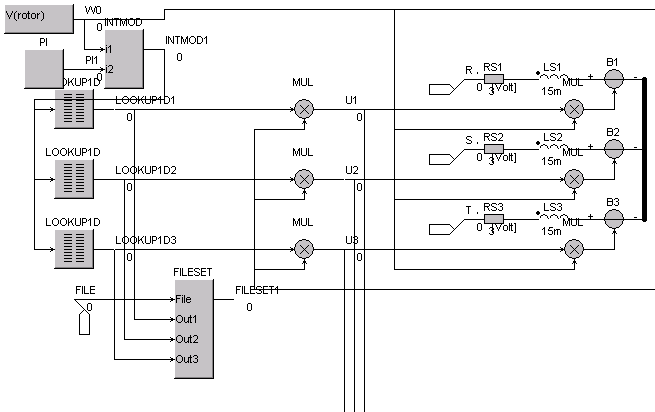Here the angular position of the rotor is calculated using the integrator block INTMOD. The angular position is the index for the LookUp1D blocks. The output from the LookUp1D blocks are the normalized back-emf voltage per phase. After multiplication with the back-emf constant k and multiplying with the angular speed w0, the back-emf is used to control the three controlled voltage sources B1, B2 and B3. The filename for the LookUp1D blocks is set by the FILESET block, wher the user can set the naem for the data file in the FILENAME block, connected to the exported label File.

The data file bldcm_fem\backemf.dat is stored in the folder bldcm_fem with the same name as the simulation file bldcm_fem.csi. We can open the data file from the Project Manager by selecting Project/File/backemf.dat.